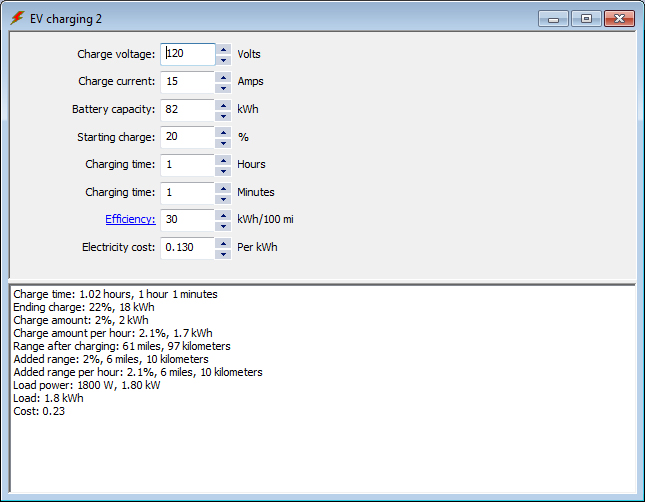## EV Charging Calculation 2

Use this calculation to determine EV charging amount.Enter your charging voltage, cuarging current, battery capacity, starting charge, charging time, efficiency, and electricity cost and calculate your charge time, total charge amount, charge amount per hour, range after charging, total added range, added range per hour, load power, load, and charging cost.

EV battery calculation

EV charging calculation 1

EV efficiency calculation

EV range calculation 1

EV range calculation 2

EV graph